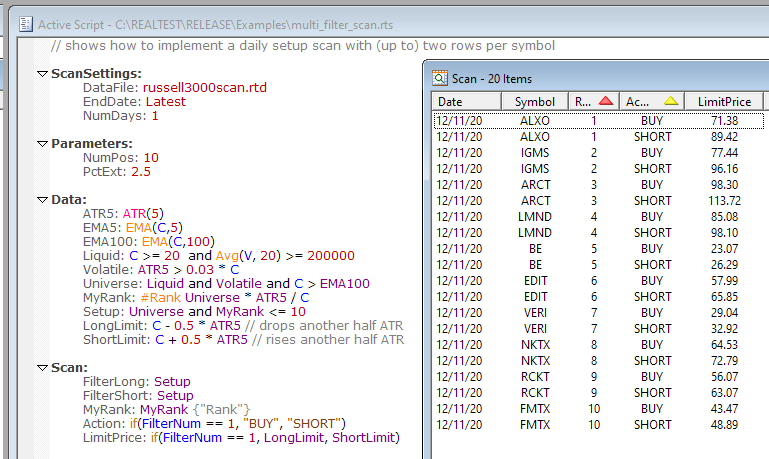By default, RealTest scans will contain, at most, one row per symbol per date. In certain cases, you might need to output multiple rows for the same symbol on the same date, with different contents. One example of this would be using a scan to generate an order list for IB Basket Trader for a set of strategies that produce more than one order for the same symbol.

To potentially output multiple rows per symbol per date, simply use multiple filter formulas. The maximum number of rows per symbol per date will be the number of different filter formulas provided. As with a single filter, row is only output for any filter if the formula returns a non-zero value ("TRUE") for that symbol on that date.

To add multiple filters to a scan definition, just start the name of each one with the word "filter" and then add one or more other characters to make their names unique. In the example below they're simply called "filter1" and "filter2", but it is not a requirement that they be numbered.

Internally, the scan processor will loop through all the dates in the scan range, then for each date it loops through all the filter formulas (or just does one loop if none is specified), then for each formula it loops through all the stocks. If the stock passes the current filter formula then all the other formulas are evaluated and a new rows is added to the scan output.

When specifying the formulas for the columns that you want to output, you will need to know which filter formula caused the current row to be included. This information is provided by the FilterNum syntax element. FilterNum simply returns the filter number currently being evaluated. The number returned refers to the sequence in which the filters were listed in the script. Any numbers that happen to be included in the filter names are ignored.

Here’s a simplified example of a long/short MR strategy pair using the same set of setups, just different limit prices, configured so each “order” would have its own scan row (some execution engines such as IB basket trader require this):Of course for actual basket order generation you’d need extra columns, etc.